Accelerate your child’s learning
Smartick is a fun way to learn math!Jul20

# Learn How to Solve Division with Decimal Numbers

In this post, we are going to learn how to solve division with decimal numbers. We will see various examples.

Finally we are going to see how to complete division problems until the remainder equals zero.

### Dividing a decimal number by a whole number

You divide as though they were whole numbers. Simply put the decimal point in the quotient location directly above its position in the dividend.

We are going to see an example, dividing 77.5 by 25.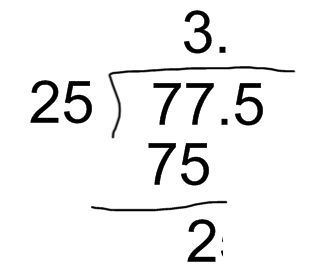77 divided by 25 equals 3, with a remainder of 2.

Now, we drop down the following digit. Seeing as the 5 is the first decimal number, we will write the decimal point in the quotient and divide 25 by 25, which equals 1.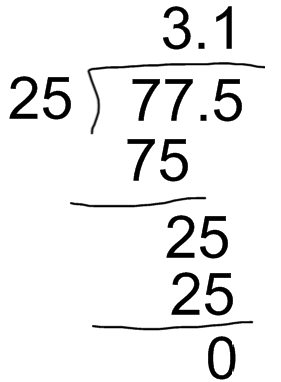1 x 25 = 25, thus 25 goes in once with no remainder.

The result of this decimal division by a whole number is 3.1 and the remainder is zero.

### Dividing a whole number by a decimal number

For example, we are going to divide 278 by 3.6.

There cannot be decimals in the divisor; therefore, remove the decimal point from the divisor and add as many zeros to the dividend as there are digits after the decimal point.

In this case, we have to add only one zero. So now we have to divide 2780 by 36.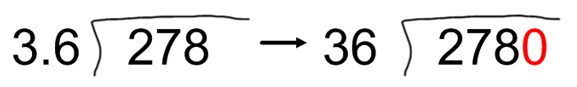36 goes into 278 seven times with a remainder of 26.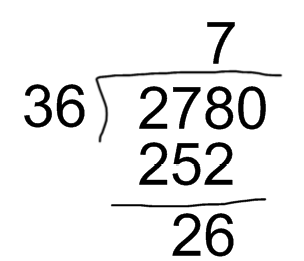Now drop down the 0, so that we can divide 260 by 36, which equals 7 with a remainder of 8.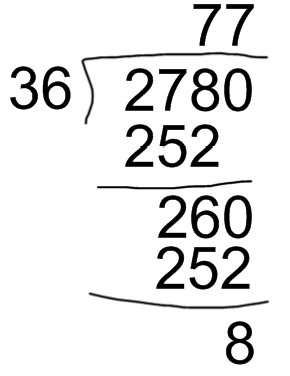The solution of the division is 77 with a remainder of 8.

### Dividing a decimal number by a decimal number

Remove the decimal point from the divisor and move the decimal point of the dividend to the right as many digits as the divisor has following the decimal point. Add zeros to the dividend as necessary.

Now we are going to divide 278.1 by 2.52.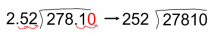First remove the decimal point from the divisor and, since the divisor has two decimals, you have to move the decimal point of the dividend two positions. If the dividend only has one decimal, add as many zeroes as there are positions left. In this case, we add one zero and we divide 27810 by 252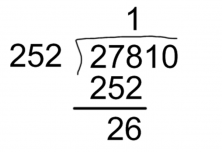252 goes into 278 once with a remainder of 26.

We drop down the following number, which is a 1, so now we have to divide 261 by 252, which goes in once, with a remainder of 9.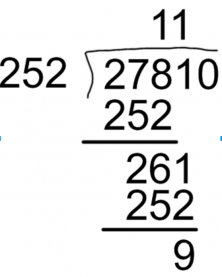We drop down the following number, which is a 0, so now we have to divide 90 by 252. Since 90 is smaller than 252, we have to write a 0 in the quotient and drop down the next digit.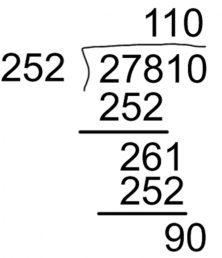We have already finished solving the division because there are no more digits. The solution would be 110 with a remainder of 90.

Now, we are going to see how to take out decimals. If we are left with a remainder at the end of the division, we write a decimal point in the quotient and we add a zero to the dividend. If we want to continue taking out decimals, we will have to keep adding zeros to the dividend.

We are going to see an example of taking out decimals, dividing 33 by 6.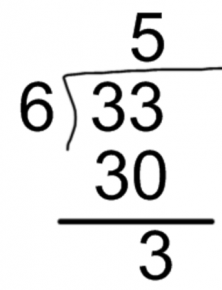#### 6 goes into 33, 5 times with a remainder of 3.

So if we want to add decimals, we have to put a decimal point in the quotient, behind the 5.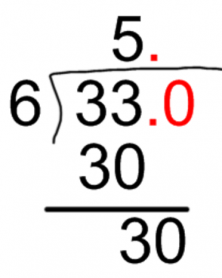Then, we can add a zero to the remainder.

Now we have to divide 30 by 6, which equals 5.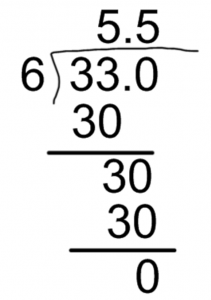And the solution of 33 divided by 6 equals 5.5.

Sign up for a free trial at Smartick today!

Fun is our brain’s favorite way of learning
Diane Ackerman
Smartick is a fun way to learn math
• 15 fun minutes a day